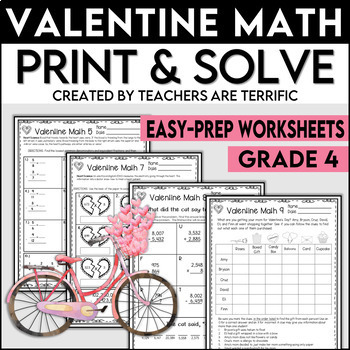# Valentine Math Print and Solve Gr. 4

Rated 5 out of 5, based on 13 reviews
13 Ratings;
4th - 5th
Subjects
Resource Type
Standards
Formats Included
• PDF
Pages
23 pages
Report this resource to TPT

### Description

February and fractions and Valentine’s Day all together with a little science thrown in, too! This set covers a lot! My math sets were invented to fill a need that you might also have! Sometimes we just need a little more practice on specific skills- especially those tricky fractions. This set takes care of that and has added features of Valentine’s day and facts about the heart!

This set of skill sheets has many more uses, too! Sub days are the perfect time to pull these out. Homework, math centers, early finishers, intervention, and more!

What makes this set unique? Each page includes scientific facts about the human heart! Win-win!

This set includes ten practice sheets covering these skills:

• Find equivalent fractions.
• Simplify fractions to thelowest terms.
• Find the lowest common denominators.
• Add fractions with unlike denominators.
• Subtract fractions with unlike denominators.
• Multiply fractions.
• Multiply whole numbers.
• Add, subtract, and multiply to solve a riddle.
• Logic Puzzle
• Challenging Variety of multi-step problems

The Worksheets:

• Some pages have a review problem and/or a bonus problem.
• The review problem and bonus problem may cover a different skill or multiple steps.
• The logic puzzle has clues to identify different which gifts were purchased for Valentine’s Day.
• The Multi-step page has five problems covering a variety of skills in a challenging word-problem format.

Answer keys are included! The multistep page has an additional answer page showing how to solve the problems.

You might also enjoy these Valentine's Day Resources:

*****************************************************************************

Be the first to know about my new discounts, freebies, and product launches:

Thank you so much,

Teachers Really are Terrific!

Total Pages
23 pages
N/A
Teaching Duration
N/A
Report this resource to TPT
Reported resources will be reviewed by our team. Report this resource to let us know if this resource violates TPT’s content guidelines.

### Standards

to see state-specific standards (only available in the US).
Fluently add and subtract multi-digit whole numbers using the standard algorithm.
Multiply a whole number of up to four digits by a one-digit whole number, and multiply two two-digit numbers, using strategies based on place value and the properties of operations. Illustrate and explain the calculation by using equations, rectangular arrays, and/or area models.
Solve multistep word problems posed with whole numbers and having whole-number answers using the four operations, including problems in which remainders must be interpreted. Represent these problems using equations with a letter standing for the unknown quantity. Assess the reasonableness of answers using mental computation and estimation strategies including rounding.
Explain why a fraction 𝘢/𝘣 is equivalent to a fraction (𝘯 × 𝘢)/(𝘯 × 𝘣) by using visual fraction models, with attention to how the number and size of the parts differ even though the two fractions themselves are the same size. Use this principle to recognize and generate equivalent fractions.
Compare two fractions with different numerators and different denominators, e.g., by creating common denominators or numerators, or by comparing to a benchmark fraction such as 1/2. Recognize that comparisons are valid only when the two fractions refer to the same whole. Record the results of comparisons with symbols >, =, or <, and justify the conclusions, e.g., by using a visual fraction model.